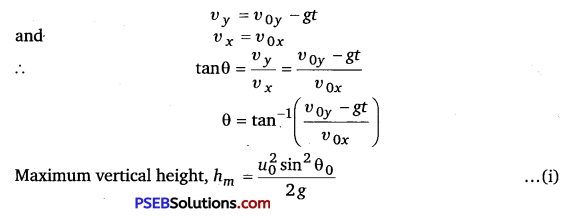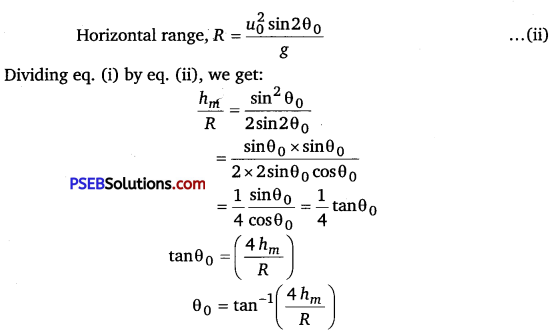# PSEB 11th Class Physics Solutions Chapter 4 Motion in a Plane

Punjab State Board PSEB 11th Class Physics Book Solutions Chapter 4 Motion in a Plane Textbook Exercise Questions and Answers.

## PSEB Solutions for Class 11 Physics Chapter 4 Motion in a Plane

PSEB 11th Class Physics Guide Motion in a Plane Textbook Questions and Answers

Question 1.
State, for each of the following physical quantities, if it is a scalar or a vector:
volume, mass, speed, acceleration, density, number of moles, velocity, angular frequency, displacement, angular velocity.
Scalar quantities: Volume, mass, speed, density, number of moles, angular frequency.
Vector quantities: Acceleration, velocity, displacement, angular velocity.

Question 2.
Pick out the two scalar quantities in the following list:
force, angular momentum, work, current, linear momentum, electric field, average velocity, magnetic moment, relative velocity.
Work and current are scalar quantities.Question 3.
Pick out the only vector quantity in the following list:
Temperature, pressure, impulse, time, power, total path length, energy, gravitational potential, coefficient of friction, charge.
Impulse is only a vector quantity in the given quantities.

Question 4.
State with reasons, whether the following algebraic operations with scalar and vector physical quantities are meaningful:
(b) adding a scalar to a vector of the same dimensions,
(c) multiplying any vector by any scalar,
(d) multiplying any two scalars,
(f) adding a component of a vector to the same vector.
(a) Not meaningful
Explanation: Adding any two scalars is not meaningful because only the scalars of same dimensions can be added.

(b) Not meaningful
Explanation: The addition of a vector quantity with a scalar quantity is not meaningful.

(c) Meaningful
Explanation: A scalar can be multiplied with a vector. For example, force is multiplied with time to give impulse.

(d) Meaningful
Explanation: A scalar, irrespective of the physical quantity it represents, can be multiplied with another scalar having the same or different dimensions.

(e) Not meaningful
Explanation: Adding any two vectors is not meaningful because only vectors of same dimensions can be added.

(f) Meaningful
Explanation: A component of a vector can be added to the same vector as they both have the same dimensions.

Question 5.
Read each statement below carefully and state with reasons, if it is true or false:
(a) The magnitude of a vector is always a scalar,
(b) each component of a vector is always a scalar,
(c) the total path length is always equal to the magnitude of the displacement vector of a particle,
(d) the average speed of a particle (defined as total path length divided by the time taken to cover the path) is either greater or equal to the magnitude of average velocity of the particle over the same interval of time,
(e) Three Vectors not lying in a plane can never add up to give a null vector.
(a) True
Explanation: The magnitude of a vector is a number. Hence, it is a scalar.

(b) False
Explanation: Each component of a vector is also a vector.

(c) False
Explanation: Total path length is a scalar quantity, whereas displacement is a vector quantity. Hence, the total path length is always greater than the magnitude of displacement. It becomes equal to the magnitude of displacement only when a particle is moving in a straight line.

(d) True
Explanation: It is because of the fact that the total path length is always greater than or equal to the magnitude of displacement of a particle.

(e) True
Explanation: Three vectors, which do not he in a plane, cannot be represented by the sides of a triangle taken in the same order.Question 6.
Establish the following vector inequalities geometrically or otherwise:
(a) $$|\vec{a}+\vec{b}| \leq|\vec{a}|+|\vec{b}|$$
(b) $$|\vec{a}+\vec{b}| \geq|| \vec{a}|-| \vec{b}||$$
(c) $$|\vec{a}-\vec{b}| \leq|\vec{a}|+|\vec{b}|$$
(d) $$|\vec{a}-\vec{b}| \geq|| \vec{a}|-| \vec{b}||$$
When does the equality sign above apply?
Solution:
(a) Let two vectors $$\vec{a}$$ and $$\vec{b}$$ be represented by the adjacent sides of a parallelogram OMNP, as shown in the given figure.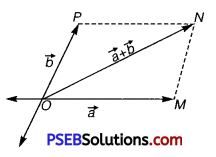$$|\overrightarrow{O M}|=|\vec{a}|$$ ……………. (i)
$$|\overrightarrow{M N}|=|\overrightarrow{O P}|=|\vec{b}|$$ ……………. (ii)
$$|\overrightarrow{O N}|=|\vec{a}+\vec{b}|$$ ……………. (iii)
In a triangle, each side is smaller than the sum of the other two sides. Therefore, in ∆ OMN, we have:
ON < (OM + MN)
$$|\vec{a}+\vec{b}|<|\vec{a}|+|\vec{b}|$$ ………….. (iv)
If the two vectors $$\vec{a}$$ and $$\vec{b}$$ act along a straight line in the same direction, then we can write: $$|\vec{a}+\vec{b}|=|\vec{a}|+|\vec{b}|$$ …………… (v)
Combining equations (iv) and (v), we get: $$|\vec{a}+\vec{b}| \leq|\vec{a}|+|\vec{b}|$$ (b) Let two vectors $$\vec{a}$$ and $$\vec{b}$$ be represented by the adjacent sides of a parallelogram OMNP, as shown in the given figure.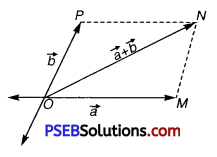Here, we have:
$$|\overrightarrow{O M}|=|\vec{a}|$$ …………….. (i)
$$|\overrightarrow{M N}|=|\overrightarrow{O P}|=|\vec{b}|$$ …………….. (ii)
$$|\overrightarrow{O N}|=|\vec{a}+\vec{b}|$$ …………….. (iii)
In a triangle, each side is smaller than the sum of the-other two sides. Therefore, in ∆ OMN, we have: ON + MN > OM
ON + OM > MN
$$|\overrightarrow{O N}|>|\overrightarrow{O M}-\overrightarrow{O P}|$$ (∵ OP = MN)
$$|\vec{a}+\vec{b}|>\| \vec{a}|-| \vec{b}||$$ ……………….. (iv)

If the two vectors $$\vec{a}$$ and $$\vec{b}$$ act along a straight line in the same direction, then we can write:
$$|\vec{a}+\vec{b}|=\|\vec{a}|-| \vec{b}\|$$ ……………. (v)
Combining equations (iv) and (v), we get:
$$|\vec{a}+\vec{b}| \geq \| \vec{a}|-| \vec{b}||$$

(c) Let two vectors $$\vec{a}$$ and $$\vec{b}$$ be represented by the adjacent sides of a parallelogram PORS, as shown in the given figure.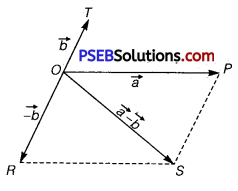Here we have:
$$|\overrightarrow{O R}|=|\overrightarrow{P S}|=|-\vec{b}|$$ ……………… (i)
$$|\overrightarrow{O P}|=|\vec{a}|$$ …………….. (ii)
$$|\overrightarrow{O S}|=|\vec{a}-\vec{b}|$$ …………….. (iii)
In a triangle, each side is smaller than the sum of the other two sides. Therefore, in ∆ OPS, we have:
OS < OP + PS
$$|\vec{a}-\vec{b}|<|\vec{a}|+|-\vec{b}|$$
$$|\vec{a}-\vec{b}|<|\vec{a}|+|\vec{b}|$$ …………… (iv)
If the two vectors act in a straight line but in opposite directions, then we can write:
$$|\vec{a}-\vec{b}|=|\vec{a}|+|\vec{b}|$$ …………….. (v)
Combining equations (iv) and (v), we get
$$|\vec{a}-\vec{b}| \leq|\vec{a}|+|\vec{b}|$$

(d) Let two vectors $$\vec{a}$$ and $$\vec{b}$$ be represented by the adjacent sides of a parallelogram PORS, as shown in the given figure.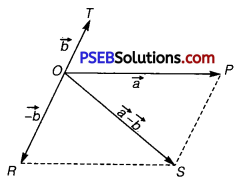The following relations can be written for the given parallelogram. OS + PS > OP …………… (i)
OS > OP – PS ……………. (ii)
$$|\vec{a}-\vec{b}|>|\vec{a}|-|\vec{b}|$$ ……………….. (iii)
The quantity on the LHS is always positive and that on the RHS can be positive or negative. To make both quantities positive, we take modulus on both sides as:
$$\|\vec{a}-\vec{b}\|>\|\vec{a}|-| \vec{b}\|$$
$$|\vec{a}-\vec{b}|>|| \vec{a}|-| \vec{b}||$$ ………………. (iv)
If the two vectors act in a straight line but in the opposite directions, then we can write:
$$|\vec{a}-\vec{b}|=\| \vec{a}|-| \vec{b}||$$ …………….(v)
Combining equations (iv) and (v), we get
$$|\vec{a}-\vec{b}| \geq|| \vec{a}|-| \vec{b}||$$Question 7.
Given $$\vec{a}+\vec{b}+\vec{c}+\vec{d}$$ = 0, which of the following statements are correct:
(a) $$\vec{a}, \vec{b}, \vec{c}$$ and $$\overrightarrow{\boldsymbol{d}}$$ must each be a null vector,
(b) The magnitude of $$(\vec{a}+\vec{c})$$ equals the magnitude of $$(\vec{b}+\vec{d})$$,
(c) The magnitude of a can never be greater than the sum of the magnitudes of $$\vec{b}, \vec{c}$$ and $$\vec{d}$$,
(d) $$\vec{b}+\vec{c}$$ must lie in the plane of $$\overrightarrow{\boldsymbol{a}}$$ and $$\overrightarrow{\boldsymbol{b}}$$ if $$$\overrightarrow{\boldsymbol{a}}$$$ and $$\overrightarrow{\boldsymbol{d}}$$ are not collinear, and in the line of $$\overrightarrow{\boldsymbol{a}}$$ and $$\overrightarrow{\boldsymbol{d}}$$, if they are collinear?
Solution:
(a) Incorrect
In order to make $$\vec{a}+\vec{b}+\vec{c}+\vec{d}$$ = 0, it is not necessary to have all the four given vectors to be null vectors. There are many other combinations which can give the sum zero.

(b) Correct
$$\vec{a}+\vec{b}+\vec{c}+\vec{d}$$ = 0
$$\vec{a}+\vec{c}=-(\vec{b}+\vec{d})$$
Taking modulus on both the sides, we get:
$$|\vec{a}+\vec{c}|=|-(\vec{b}+\vec{d})|=|\vec{b}+\vec{d}|$$
Hence, the magnitude of ($$\vec{a}+\vec{c}$$) is the same as the magnitude of ($$\vec{b}+\vec{d}$$).

(c) Correct $$\vec{a}+\vec{b}+\vec{c}+\vec{d}$$ = 0
$$\vec{a}=(\vec{b}+\vec{c}+\vec{d})$$

Taking modulus on both sides, we get
$$|\vec{a}|=|\vec{b}+\vec{c}+\vec{d}|$$
$$|\vec{a}| \leq|\vec{a}|+|\vec{b}|+|\vec{c}|$$ ………………. (i)

Equation (i) shows that the magnitude of a is equal to or less than the sum of the magnitudes of $$\vec{b}$$, $$\vec{c}$$ and $$\vec{d}$$.
Hence, the magnitude of vector a can never be greater than the sum of the magnitudes of $$\vec{b}$$, $$\vec{c}$$ and $$\vec{d}$$.

(d) Correct For $$\vec{a}+\vec{b}+\vec{c}+\vec{d}$$ = 0
$$\vec{a}+(\vec{b}+\vec{c})+\vec{d}$$ = 0
The resultant sum of the three vectors $$\vec{a},(\vec{b}+\vec{c})$$ and $$\vec{d}$$ can be zero
only if ($$\vec{b}+\vec{c}$$) lie in a plane containing a and d, assuming that these
three vectors are represented by the three sides of a triangle.

If a and d are collinear/ then it implies that the vector ($$\vec{b}+\vec{c}$$ ) is in the line of $$\vec{a}$$ and $$\vec{d}$$. This implication holds only then the vector sum of all the vectors will be zero.

Question 8.
Three girls skating on a circular ice ground of radius 200m start from a point P on the edge of the ground and reach a point Q diametrically opposite to P following different paths as shown in figure below. What is the magnitude of the displacement vector for each? For which girl is this equal to the actual length of the path skate?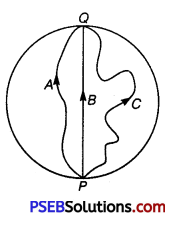Solution:
Displacement is given by the minimum distance between the initial and final positions of a particle. In the given case, all the girls start from point P and reach point Q. The magnitudes of their displacements will be equal to the diameter of the ground.
Radius of the ground = 200 m
Diameter of the ground = 2 × 200 = 400 m
Hence, the magnitude of the displacement for each girl is 400 m. This is equal to the actual length of the path skated by girl B.Question 9.
A cyclist starts from the centre O of a circular park of radius 1 km, reaches the edge P of the park, then cycles along the circumference, and returns to the centre along QO as shown in figure below. If the round trip takes 10 min, what is the (a) net displacement, (b) average velocity, and (c) average speed of the cyclist?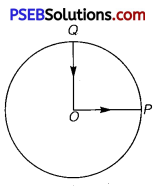Solution:
(a) Displacement is given by the minimum distance between the initial and final positions of a body. In the given case, the cyclist comes to the starting point after cycling for 10 minutes. Hence, his net displacement is zero.

(b) Average velocity is given by the relation;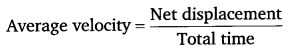Since the net displacement of the cyclist is zero, his average velocity will also be zero.

(c) Average speed of the cyclist is given by the relation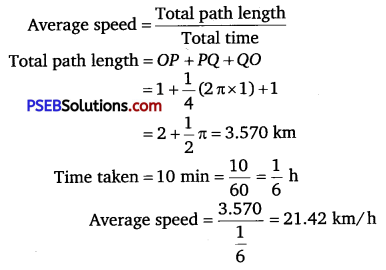Question 10.
On an open ground, a motorist follows a track that turns to his left by an angle of 60° after every 500 m. Starting from a given turn, specify the displacement of the motorist at the third, sixth and eighth turn. Compare the magnitude of the displacement with the total path length covered by the motorist in each case.
Solution:
The path followed by the motorist is a regular hexagon with side 500 m, as shown in the given figure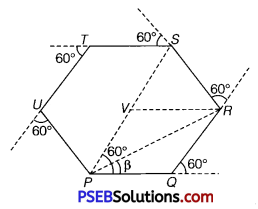Let the motorist start from point P.
The motorist takes the third turn at S.
∴ Magnitude of displacement PS = PV + VS = 500 + 500 = 1000 m
Total path length = PQ + QR +RS = 500 + 500 + 500 = 1500 m
The motorist takes the sixth turn at point P, which is the starting point.
∴ Magnitude of displacement = 0
Total path length = PQ + QR + RS + ST +TU + UP
= 500 + 500 + 500 + 500 + 500 + 500 = 3000 m
The motorist takes the eight turn at point R
∴ Magnitude of displacement = PR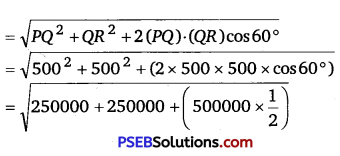= 866.03 m
If it is inclined at an angle β from the direction of PQ, then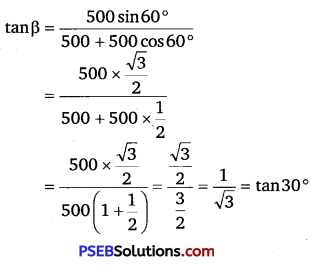or β = 30°
Therefore, the magnitude of displacement is 866.03 m at an angle of 30° with PR.
Total path length = Circumference of the hexagon + PQ + QR
= 6 × 500 + 500 + 500 = 4000 m
The magnitude of displacement and the total path length corresponding to the required turns is shown in the given

 Turn Magnitude of dispalcement (m) Total path length (m) Third 1000 1500 Sixth 0 3000 Eighth 866.03; 30° 4000

Comparison of the magnitude of displacement with the total path length in each case: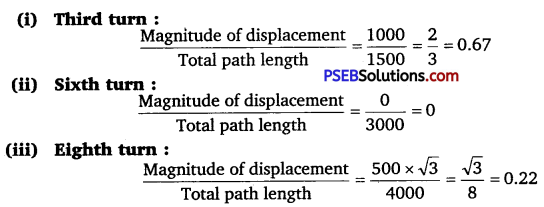Question 11.
A passenger arriving in a new town wishes to go from the station to a hotel located 10 km away on a straight road from the station. A dishonest cabman takes him along a circuitous path 23 km long and reaches the hotel in 28 min. What is (a) the average speed of the taxi, (b) the magnitude of average velocity? Are the two equal?
Solution:
Total distance travelled = 23 km
Total time taken = 28 min = $$\frac{28}{60}$$ h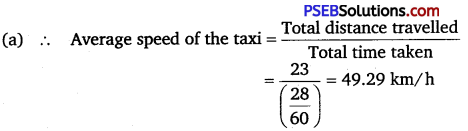(b) Distance between the hotel and the station =10 km = Displacement of the taxi
∴ Average velocity = $$\frac{\frac{10}{28}}{\frac{60}{60}}$$ = 21.43 km/ h

Therefore, the two physical quantities (average speed and average velocity) are not equal.

Question 12.
Rain is falling vertically with a speed of 30 m s-1. A woman rides a bicycle with a speed of 10 ms-1 in the north to south direction. What is the direction in which she should hold her umbrella?
Solution:
The described situation is shown in the given figure.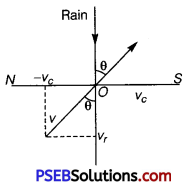Here, υc = Velocity of the cyclist
υr = Velocity of falling rain
In order to protect herself from the rain, the woman must hold her umbrella in the direction of the relative velocity (v) of the rain with respect to the woman.
υ = υr + (-υc)
= 30 + (-10) = 20 m/s
tanθ = $$\frac{v_{c}}{v_{r}}=\frac{10}{30}$$
θ = tan-1 ($$\frac{1}{3}$$)
= tan-1 (0.333) ≈ 18°
Hence, the woman must hold the umbrella toward the south, at an angle of nearly 18° with the vertical.

Question 13.
A man can swim with a speed of 4.0 km/h in still water. How long does he take to cross a river 1.0 km wide if the river flows steadily at 3.0 km/h and he makes his strokes normal to the river current? How far down the river does he go when he reaches the other hank?
Solution: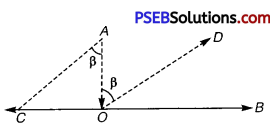Speed of the man, υm = 4 km/h
Width of the river = 1 km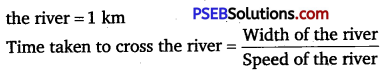= $$\frac{1}{4}$$ h = $$\frac{1}{4}$$ × 60 = 15 min
Speed of the river, υr = 3 km/h
Distance covered with flow of the river = υr × t
= 3 × $$\frac{1}{4}$$ = $$\frac{3}{4}$$ km
= $$\frac{3}{4}$$ × 1000 = 750 mQuestion 14.
In a harbour, wind is blowing at the speed of 72 km/h and the flag on the mast of a boat anchored in the harbour flutters along the N-E direction. If the boat starts moving at a speed of 51 km/h to the north, what is the direction of the flag on the mast of the boat?
Solution:
Velocity of the boat, υb = 51 km/h
Velocity of the wind, υw = 72 km/h
The flag is fluttering in the north-east direction. It shows that the wind is blowing toward the north-east direction. When the ship begins sailing toward the north, the flag will move along the direction of the relative velocity (υwb) of the wind with respect to the boat.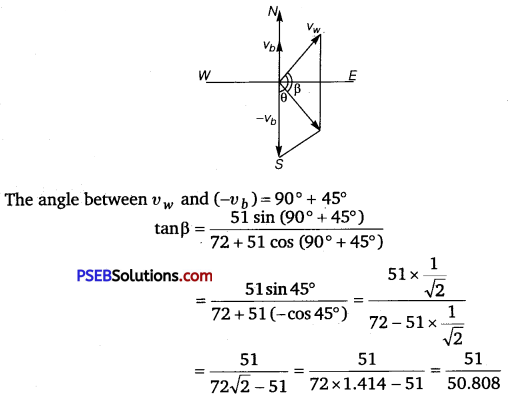∴ β = tan-1 (1.0038) = 45.18°
Angle with respect to the east direction = 45.18° – 45° = 0.18°
Hence, the flag will flutter almost due east.

Question 15.
The ceiling of a long hall is 25 m high. What is the maximum horizontal distance that a ball thrown with a speed of 40 m s 1 can go without hitting the ceiling of the hall?
Solution:
Speed of the ball, u = 40 m/s
Maximum height, h = 25 m
In projectile motion, the maximum height reached by a body projected at an angle 0, is given by the relation: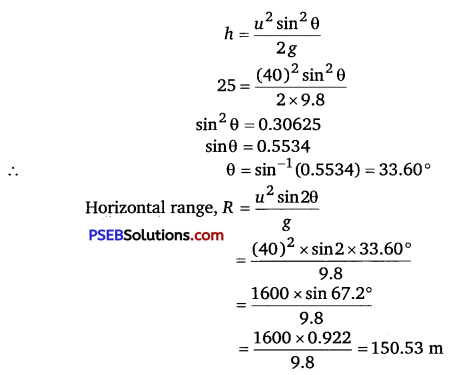Question 16.
A cricketer can throw a ball to a maximum horizontal distance of 100 m. How much high above the ground can the cricketer throw the same ball?
Solution:
Maximum horizontal distance, R = 100 m
The cricketer will only be able to throw the ball to the maximum horizontal distance when the angle of projection is 45°, i.e., θ = 45°.
The horizontal range for a projection velocity v, is given by the relation:
R = $$\frac{u^{2} \sin 2 \theta}{g}$$
100 = $$\frac{u^{2}}{g}$$ sin90°
$$\frac{u^{2}}{g}$$ = 100 ……………… (i)
The ball will achieve the maximum height when it is thrown vertically upward. For such motion, the final velocity v is zero at the maximum height H.
Acceleration, a = -g
Using the third equation of motion:
υ2 – u2 = -2gH
H = $$\frac{1}{2}$$ × $$\frac{u^{2}}{g}$$ = $$\frac{1}{2}$$ × 100 = 50 m

Question 17.
A stone tied to the end of a string 80 cm long is whirled in a horizontal circle with a constant speed. If the stone makes 14 revolutions in 25 s, what is the magnitude and direction of acceleration of the stone?
Solution:
Length of the string, l = 80 cm = 0.8 m
Number of revolutions = 14
Time taken = 25 s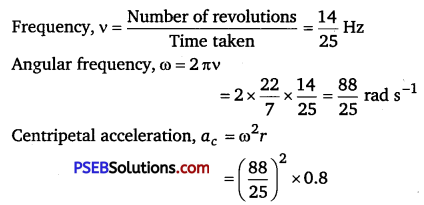= 9.91 m/s2
The direction of centripetal acceleration is always directed along the string, toward the centre, at all points.Question 18.
An aircraft executes a horizontal loop of radius 1.00 km with a steady speed of 900 km/h. Compare its centripetal acceleration with the acceleration due to gravity.
Solution:
Radius of the loop, r = 1 km = 1000 m
Speed of the aircraft,υ = 900 km/h = 900 × $$\frac{5}{18}$$ = 250 m/s
Centripetal acceleration, ac = $$\frac{v^{2}}{r}$$
= $$\frac{(250)^{2}}{1000}$$ = 62.5 m/s2
Acceleration due to gravity, g = 9.8 m/s2
$$\frac{a_{c}}{g}=\frac{62.5}{9.8}$$ = 6.38
ac = 6.38 g

Question 19.
Read each statement below carefully and state, with reasons, if it is true or false:
(a) The net acceleration of a particle in circular motion is always along the radius of the circle towards the centre.
(b) The velocity vector of a particle at a point is always along the tangent to the path of the particle at that point.
(c) The acceleration vector of a particle in uniform circular motion averaged over one cycle is a null vector.
(a) False
Reason: The net acceleration of a particle in circular motion is not always directed along the radius of the circle towards the centre. It happens only in the case of uniform circular motion.

(b) True
Reason: At a point on a circular path, a particle appears to move tangentially to the circular path. Hence, the velocity vector of the particle is always along the tangent at a point.

(c) True
Reason: In uniform circular motion (UCM), the direction of the acceleration vector points toward the centre of the circle. However, it constantly changes with time. The average of these vectors over one cycle is a null vector.

Question 20.
The position of a particle is given by
r̂ = 3.0 t î – 2.0 t2ĵ + 4.0k̂ m
where t is in seconds and the coefficients have the proper units for r to be in metres.
(a) Find the υ and a of the particle?
(b) What is the magnitude and direction of velocity of the particle at t = 3.0 s?
Solution: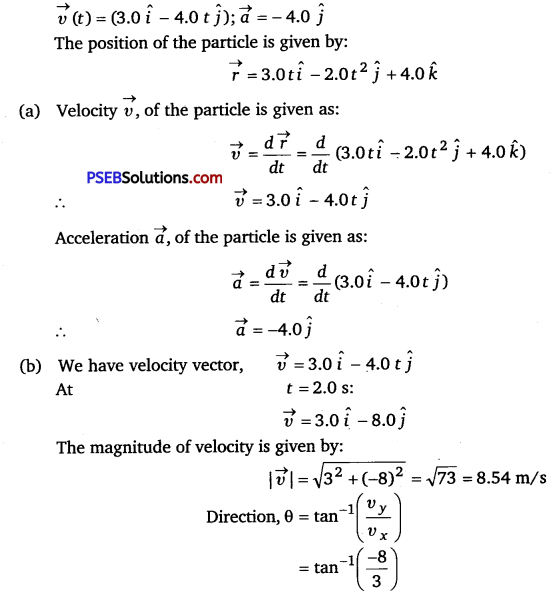= – tan-1 (2.667)
= -69.45°
The negative sign indicates that the direction of velocity is below the x-axis.Question 21.
A particle starts from the origin at t 0 s with a velocity of 10.0 ĵ m/s and moves in the x – y plane with a constant acceleration of (8.0î + 2.0ĵ) ms-2.
(a) At what time is the x – coordinate of the particle 16 m? What is the y-coordinate of the particle at that time?
(b) What is the speed of the particle at the time?
Solution: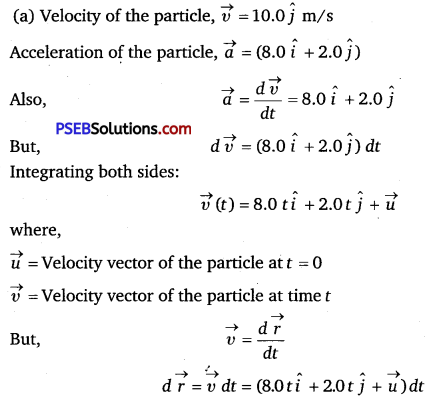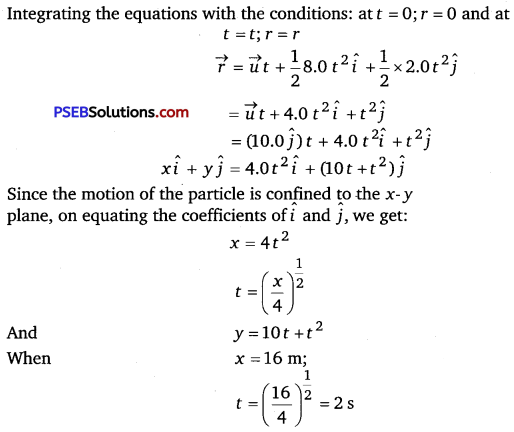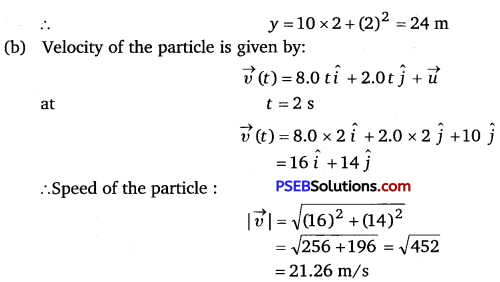Question 22.
î and ĵ are unit vectors along x- and y-axis respectively. What is the magnitude and direction of the vectors î + ĵ and î – ĵ? What are the components of a vector $$\overrightarrow{\boldsymbol{A}}$$ = 2î + 3ĵ along the directions of î + ĵ and î – ĵ? [You may use graphical method]
Solution:
Consider a vector $$\vec{P}$$, given as:
$$\vec{P}$$ = î + ĵ
Pxî +Py ĵ = î + ĵ
On comparing the components on both sides, we get:
Px = Py = 1
$$|\vec{P}|=\sqrt{P_{x}^{2}+P_{y}^{2}}=\sqrt{1^{2}+1^{2}}=\sqrt{2}$$ …………… (i)
Hence, the magnitude of the vector î + ĵ is √2.
Let 0 be the angle made by the vector , with the x-axis, as shwon in the following figure.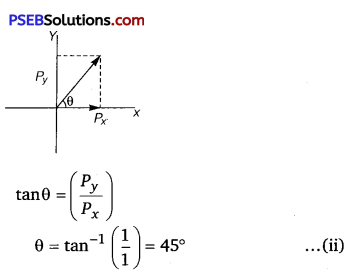Hence, the vector î + ĵ makes an angle of 45° with the x-axis.
Let $$\vec{Q}$$ = î – ĵ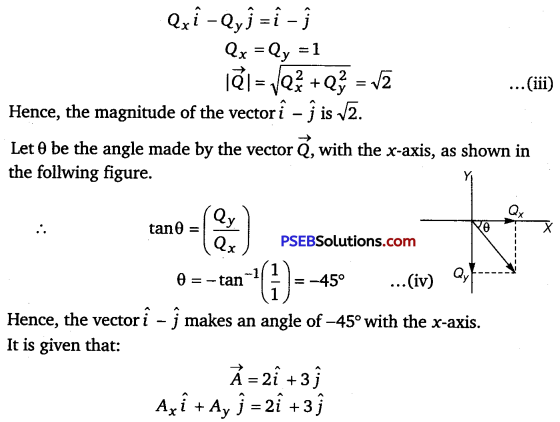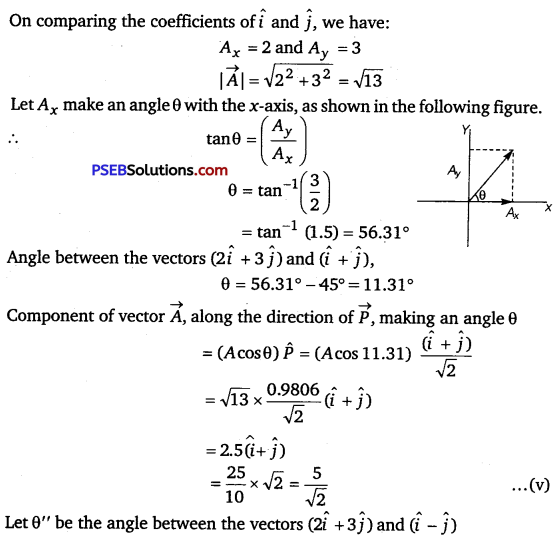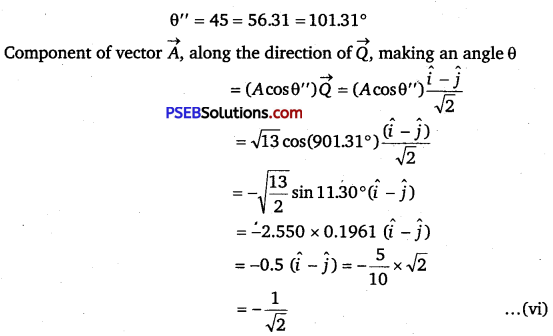Question 23.
For any arbitrary motion in space, which of the following relations are true: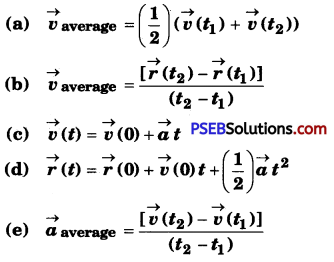(The ‘average’ stands for average of the quantity over the time interval t1 to t2)
Solution:
(b) and (e)
(a) It is given that the motion of the particle is arbitrary. Therefore, the average velocity of the particle cannot be given by this equation.
(b) The arbitrary motion of the particle can be represented by this equation.
(c) The motion of the particle is arbitrary. The acceleration of the particle may also be non-uniform. Hence, this equation cannot represent the motion of the particle in space.
(d) The motion of the particle is arbitrary; acceleration of the particle may also be non-uniform. Hence, this equation cannot represent the motion of particle in space.
(e) The arbitrary motion of the particle can be represented by this equation.

Question 24.
Read each statement below carefully and state, with reasons and examples, if it is true or false:
A scalar quantity is one that
(a) is conserved in a process
(b) can never take negative values
(c) must be dimensionless
(d) does not vary from one point to another in space
(e) has the same value for observers with different orientations of axes.
(a) False
Reason: Despite being a scalar quantity, energy is not conserved in inelastic collisions.

(b) False
Reason: Despite being a scalar quantity, temperature can take negative values.

(c) False
Reason: Total path length is a scalar quantity. Yet it has the dimension of length.

(d) False
Reason: A scalar quantity such as gravitational potential can vary from one point to another in space.

(e) True
Reason: The value of a scalar does not vary for observers with different orientations of axes.

Question 25.
An aircraft is flying at a height of 3400 m above the ground. If the angle subtended at a ground observation point by the aircraft positions 10.0 s apart is 30°, what is the speed of the aircraft?
Solution:
The positions of the observer and the aircraft are shown in the given figure.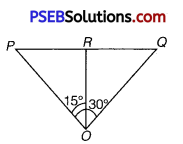Height of the aircraft from ground, OR = 3400 m
Angle subtended between the positions, ∠POQ =30°
Time = 10 s
In Δ PRO:
tan15° = $$\frac{P R}{O R}$$
PR = OR tan 15°
= 3400 × tan15°
Δ PRO is similar to Δ RQO.
PR =RQ
Motion in a Plane 81
PQ = PR + RQ
= 2PR = 2 × 3400 tanl5°
= 6800 × 0.268 = 1822.4 m
speed of the aircraft = $$\frac{1822.4}{10}$$ = 182.24 m/sQuestion 26.
A vector has magnitude and direction. Does it have a location in space? Can it vary with time? Will two equal vectors a and b at different locations in space necessarily have identical physical ‘ effects? Give examples in support of your answer.
No; Yes; No
Generally speaking, a vector has no definite locations in space. This is because a vector remains invariant when displaced in such a way that its magnitude and direction remain the same. However, a position vector has a definite location in space.

A vector can vary with time. For example, the displacement vector of a particle moving with a certain velocity varies with time.

Two equal vectors located at different locations in space need not produce the same physical effect. For example, two equal forces acting on an object at different points can cause the body to rotate, but their combination cannot produce an equal turning effect.

Question 27.
A vector has both magnitude and direction. Does it mean that anything that has magnitude and direction is necessarily a vector? The rotation of a body can be specified by the direction of the axis of rotation, and the angle of rotation about the axis. Does that make any rotation a vector?
No; No
A physical quantity having both magnitude and direction need not be considered a vector. For example, despite having magnitude and direction, current is a scalar quantity. The essential requirement for a physical quantity to be considered a vector is that it should follow the law of vector addition.

Generally speaking, the rotation of a body about an axis is not a vector quantity as it does not follow the law of vector addition. However, a rotation by a certain small angle follows the law of vector addition and is therefore considered a vector.

Question 28.
Can you associate vectors with (a) the length of a wire bent into a loop, (b) a plane area, (c) a sphere? Explain.
No; Yes; No
One cannot associate a vector with the length of a wire bent into a loop. One can associate an area vector with a plane area. The direction of this vector is normal, inward or outward to the plane area.
One cannot associate a vector with the volume of a sphere. However, an area vector can be associated with the area of a sphere.Question 29.
A bullet fired at an angle of 30° with the horizontal hits the ground 3.0 km away. By adjusting its angle of projection, can one hope to hit a target 5.0 km away? Assume the muzzle speed to the fixed, and neglect air resistance.
Solution:
No
Range, R = 3 km
Angle of projection, θ = 30°
Acceleration due to gravity, g = 9.8 m/s2
Horizontal range for the projection velocity u0, is given by the relation :
R = $$\frac{u_{0}^{2} \sin 2 \theta}{g}$$
3 = $$\frac{u_{0}^{2}}{g}$$ sin 60°
$$\frac{u_{0}^{2}}{g}$$ = 2√3 ……………… (i)
The maximum range (Rmax) is achieved by the bullet when it is fired at an angle of 45° with the horizontal, that is,
Rmax = $$\frac{u_{0}^{2}}{g}$$ = ………………. (ii)
On comparing equations (i) and (ii), we get:
Rmax = 2√3 × 1.732 = 3.46 km
Hence, the bullet will not hit a target 5 km away.

Question 30.
A fighter plane flying horizontally at an altitude of 1.5 km with speed 720 km/h passes directly overhead an dnti-aircraft gun. At what angle from the vertical should the gun be fired for the shell with muzzle speed 600 ms-1 to hit the plane? At what minimum altitude should the pilot fly the plane to avoid being hit? (Take g = 10ms-2)
Solution:
Height of the fighter plane = 1.5 km = 1500 m
Speed of the fighter plane, υ = 720 km/h = 200 m/s
Let θ be the angle with the vertical so that the shell hits the plane. The
situation is shown in the given figure.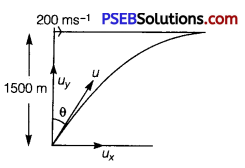Muzzle velocity of the gun, u = 600 m/s
Time taken by the shell to hit the plane = t
Horizontal distance travelled by the shell = uxt
Distance travelled by the plane = υt
The shell hits the plane. Hence, these two distances must be equal.
uxt = υt
usinθ = υ
sinθ = $$\frac{v}{u}=\frac{200}{600}=\frac{1}{3}$$ 0.33
θ = sin-1 (0.33) = 19.5°
In order to avoid being hit by the shell, the pilot must fly the plane at
an altitude (H) higher than the maximum height achieved by the shell.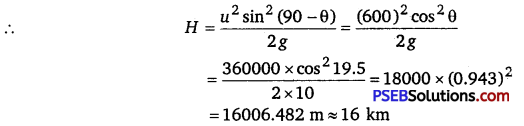Question 31.
A cyclist is riding with a speed of 27 km/h. As he approaches a circular turn on the road of radius 80 m, he applies brakes and reduces his speed at the constant rate of 0.50 m/s every second. What is the magnitude and direction of the net acceleration of the cyclist on the circular turn?
Solution:
Speed of the cyclist, υ = 27 km/h = 7.5 m/s
Radius of the circular turn , r = 80m
Centripetal acceleration is given as:
a = $$\frac{v^{2}}{r}$$
= $$\frac{(7.5)^{2}}{80}$$ = 0.7 m/s2
The situation is shown in the given figure: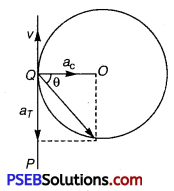Suppose the cyclist begins cycling from point P and moves toward point Q. At point Q, he applies the brakes and decelerates the speed of the bicycle by 0.5 m/s2.
This acceleration is along the tangent at Q and opposite to the direction of motion of the cyclist.
Since the angle between ac and aT is 90°, the resultant acceleration a is given by:
a = $$\sqrt{a_{c}^{2}+a_{T}^{2}}=\sqrt{(0.7)^{2}+(0.5)^{2}}=\sqrt{0.74}$$ = 0.86 m/s2
and
tan θ = $$\frac{a_{c}}{a_{T}}$$
where θ is the angle of the resultant with the direction of velocity
tanθ = $$\frac{0.7}{0.5}$$ = 1.4
θ = tan-1 (1.4) = 54.46°
Hence, the net acceleration of the cyclist is 0.86 rn/s2, 54.60 0 with the direction of velocity.Question 32.
(a) Show that for a projectile the angle between the velocity and the x-axis as a function of time is
θ(t) = tan ($$\frac{v_{0 y}-g t}{v_{0 x}}$$)

(b) Show that the projection angle θ0 for a projectile launched from the origin is given by
θ0 = tan-1 ($$\frac{\mathbf{4} \boldsymbol{h}_{\boldsymbol{m}}}{\boldsymbol{R}}$$)
where the symbols have their usual meaning.
Solution:
Let y Ox and y 0, respectively be the initial components of the velocity of the projectile along horizontal (x) and vertical (y) directions.
Let y and y , respectively be the horizontal and vertical components of velocity at a point P.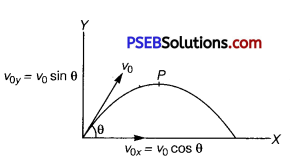Time taken by the projectile to reach point P = t.
Applying the first equation of motion along the vertical and horizontal directions, we get: